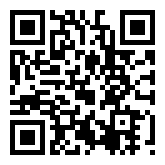# 在Python中用PIL做验证码

1. 生成一个固定大小的白色图片。
2. 在图片上随机写几个字母。

# 1. 基本操作

# -*- coding: utf-8 -*-

from PIL import Image

im = Image.new('1', (100, 100), 'white')
im.show()


Imagenew 方法新创建一个图片对象，第一个参数指定“模式”，不同的模式对应的每个像素的颜色表示也不同。比如：

• 1 按文档说是单色模式，但事实上它和 L 一样，是灰度模式。
• L 灰度模式，每个像素的颜色使用 0-255 的整数表示。
• RGBA 三元色加透明度的表示方式，每个像素的颜色使用类似 (12,34,23,1) 的 tuple 表示。

new 的第二个参数指定图片的大小，第三个参数指定背影色。

show 方法是使用系统提供的工具把图片马上显示出来。

# -*- coding: utf-8 -*-

from PIL import Image
from PIL import ImageDraw

im = Image.new('1', (100, 100), 'white')
draw = ImageDraw.Draw(im)
draw.text((0, 0), 'hello world!')
im.show()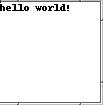pilfont.py  xxx.pcf


# -*- coding: utf-8 -*-

from PIL import Image
from PIL import ImageDraw
from PIL import ImageFont

im = Image.new('1', (100, 100), 'white')
draw = ImageDraw.Draw(im)
draw.text((0, 0), 'hello world!', font=FONT)
im.show()font = ImageFont.truetype("arial.ttf", 15)


# 2. 加入变化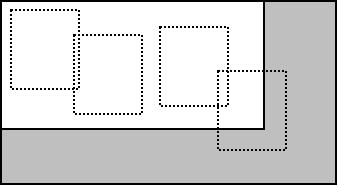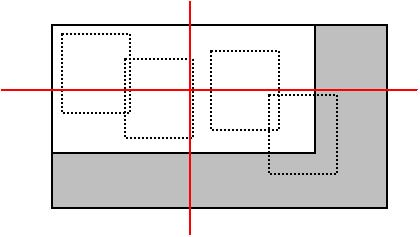# -*- coding: utf-8 -*-

import PIL
from PIL import Image
from PIL import ImageDraw

im = Image.new('1', (500, 500), 'white')
draw = ImageDraw.Draw(im)
draw.line(((0, 0), (100, 200)))
im.show()


# 3. 最后完成

fileio = StringIO()
im.save(fileio, 'gif')
im.show()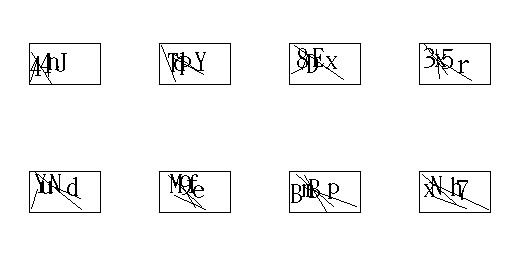# 4. 代码

# -*- coding: utf-8 -*-
#AUTHOR: yeshengzou # # gmail.com
#DATE: 2012.4.23
#LICENCE: GPLv3

import PIL
from PIL import Image
from PIL import ImageDraw
from PIL import ImageFont
from random import randint
from cStringIO import StringIO

CHAR = 'acdefghijkmnpqrstuvwxyABCDEFGHJKLMNPQRSTUVWXY345789'
LEN = len(CHAR) - 1
X_SPACE = 6 #两个字符之间最少相隔多少个像素
TRY_COUNT = 30 #随机字符的位置尝试最多多少次,避免死循环
WIDTH = 70
HEIGHT = 40

def gen():
im = Image.new('1', (WIDTH, HEIGHT), 'white')
draw = ImageDraw.Draw(im)
w, h = im.size

#S = [(x, y, 'c')]
S = []
x_list = []
y_list = []
n = 0
while True:
n += 1
if n > TRY_COUNT:
break
x = randint(0, w - PADDING)
flag = True
for i in x_list:
if abs(x - i) < X_SPACE:
flag = False
continue
if not flag:
break
if not flag:
continue

y = randint(0, h - PADDING)
x_list.append(x)
y_list.append(y)
S.append((x, y, CHAR[randint(0, LEN)]))
if len(S) == 4:
break

for x, y, c in S:
draw.text((x, y), c, font=FONT)

#加3根干扰线
for i in range(3):
x1 = randint(0, (w - PADDING) / 2)
y1 = randint(0, (h- PADDING / 2))
x2 = randint(0, w)
y2 = randint((h - PADDING / 2), h)
draw.line(((x1, y1), (x2, y2)), fill=0, width=1)

S.sort(lambda x, y: 1 if x > y else -1)
char = [x for x in S]
fileio = StringIO()
im.save(fileio, 'gif')
im.show()
return ''.join(char), fileio

if __name__ == '__main__':
print gen()